Chapter 3.5, Problem 64E

Chapter
Section
Textbook Problem

# In Exercises 59–64, match the graph of f to the graph of f ′ . (The graphs of f ' are shown after Exercise 64.)Graph of derivatives for Exercises 59-64:(A)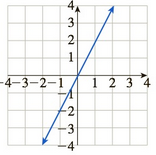(B)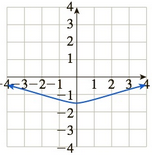(C)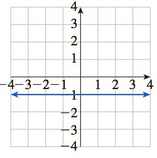(D)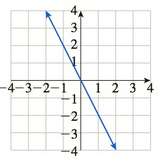(E)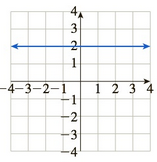(F)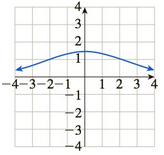To determine

The graph of f of f from the provided graphs.

The graph of f is: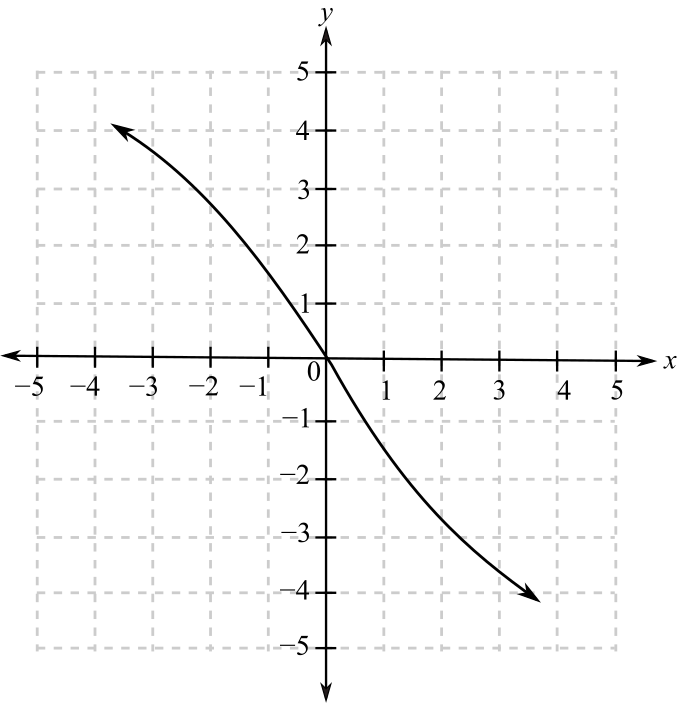The graphs provided for f are:Explanation

Given Information:

The graph of f is:

The graphs provided for f are:

When a function is increasing or rising, the slope of the function is positive and greatest where the tangent is more slanted whereas when the function is decreasing or falling, its slope is negative and it has the least value where the tangent is the most slanted

### Still sussing out bartleby?

Check out a sample textbook solution.

See a sample solution

#### The Solution to Your Study Problems

Bartleby provides explanations to thousands of textbook problems written by our experts, many with advanced degrees!

Get Started

## Additional Math Solutions

#### Find more solutions based on key concepts